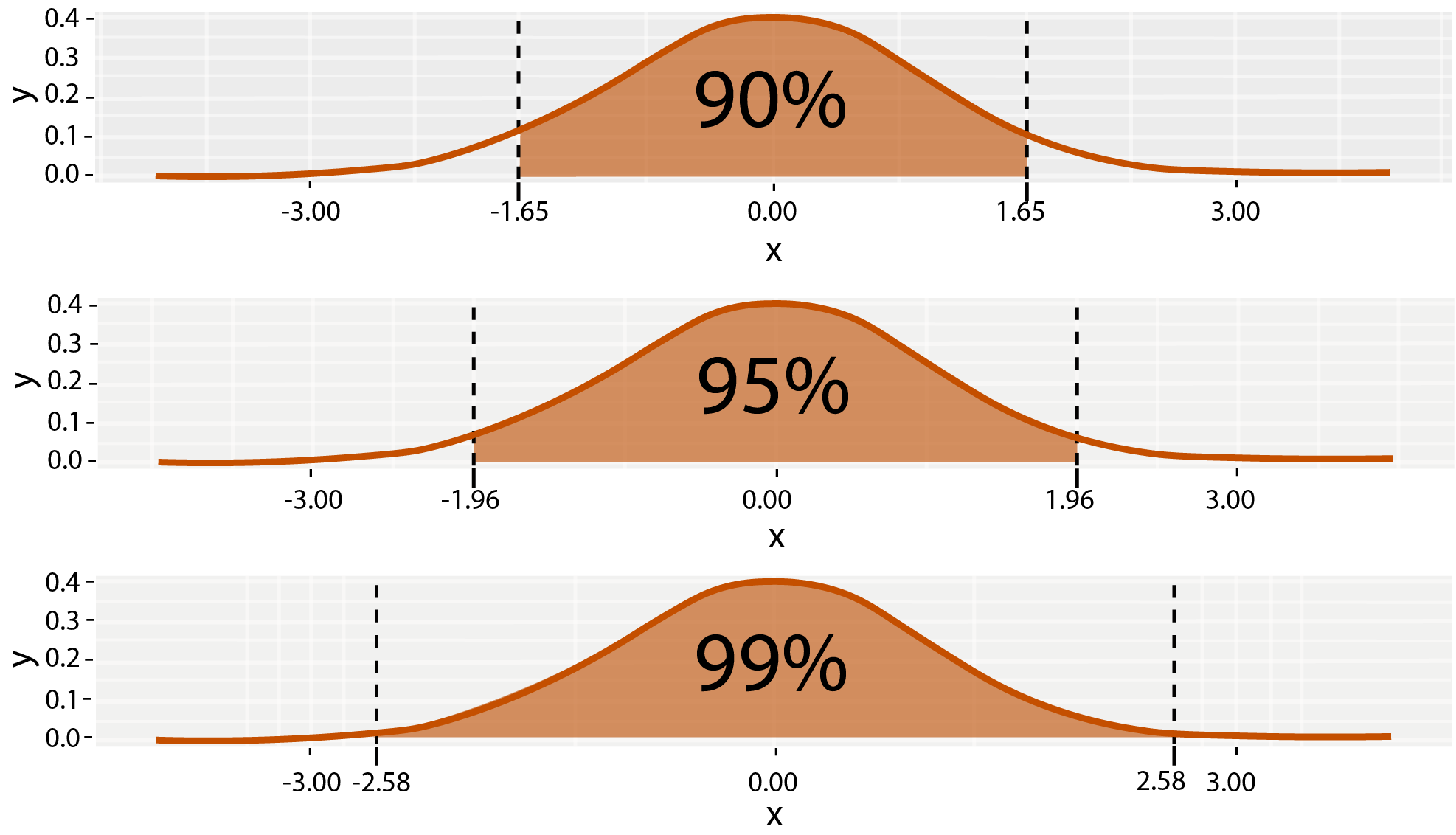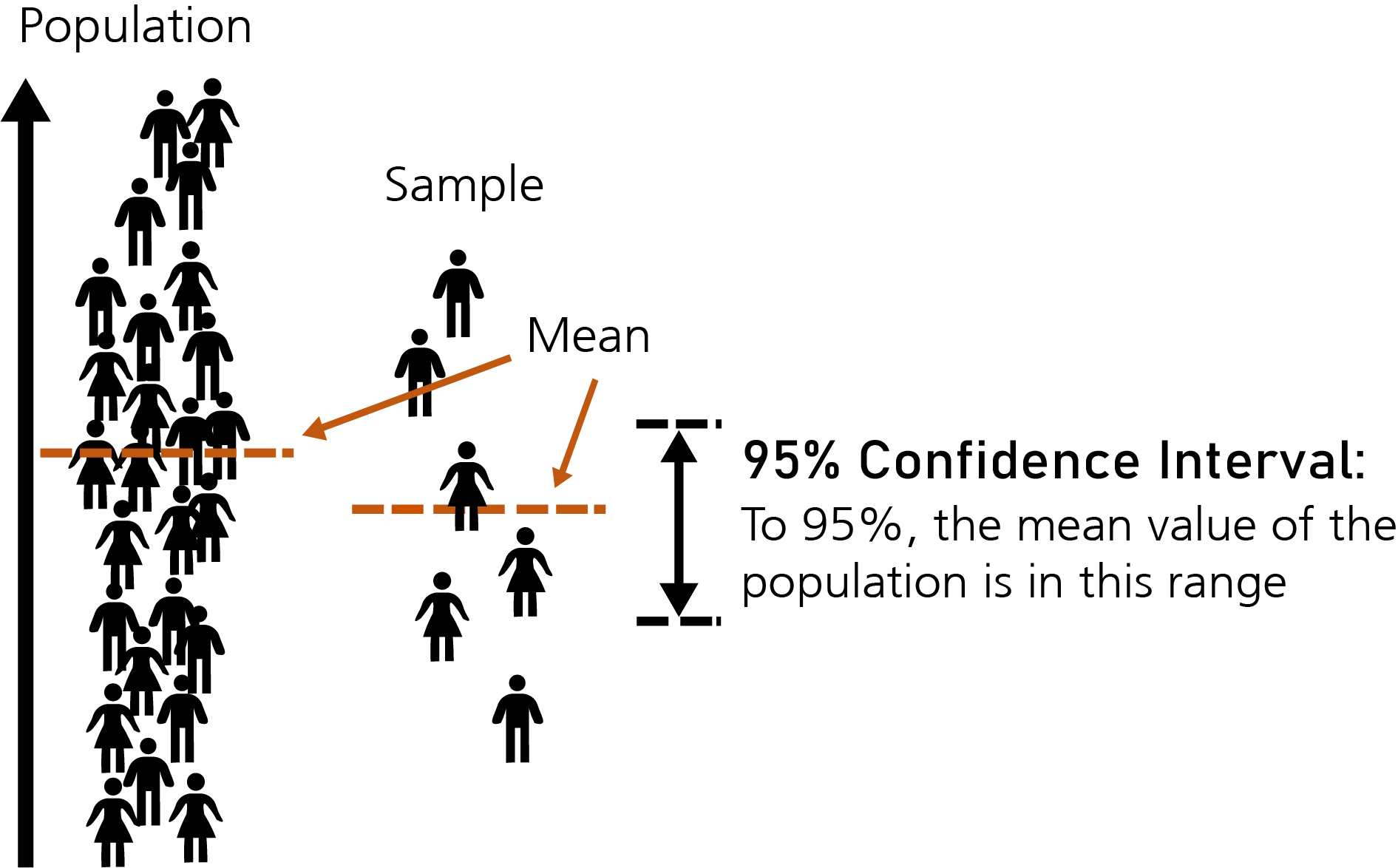Course Content

# Learning Statistics with Python

Learning Statistics with Python

##Confidence Interval

What is a Confidence Interval?

A confidence interval is a range of estimated values for an unknown parameter. To calculate it, we need to specify the confidence level. The most common confidence level is 95%, but 90% and 99% confidence levels are also used.

What Does a 95% Confidence Level Mean?

A 95% confidence level means that in 95% of cases, the estimate will fall within the lower and upper bounds of the interval.Alpha Value

The confidence interval is typically equal to 1 minus alpha (1-a), where alpha represents the probability of a Type I error. Type I errors occur when we incorrectly reject a true null hypothesis.

Null and Alternative Hypotheses

The null and alternative hypotheses are assumptions used in statistical tests to make judgments based on data. In statistical hypothesis testing, the null and alternative hypotheses are mutually exclusive statements. Generally, in hypothesis testing, we either try to reject or fail to reject the null hypothesis.

In this section, we will work with penguin data. By the end of this section, we will confidently state that with a 95% level of confidence, the mean penguin mass is...

Everything was clear?

Section 5. Chapter 2